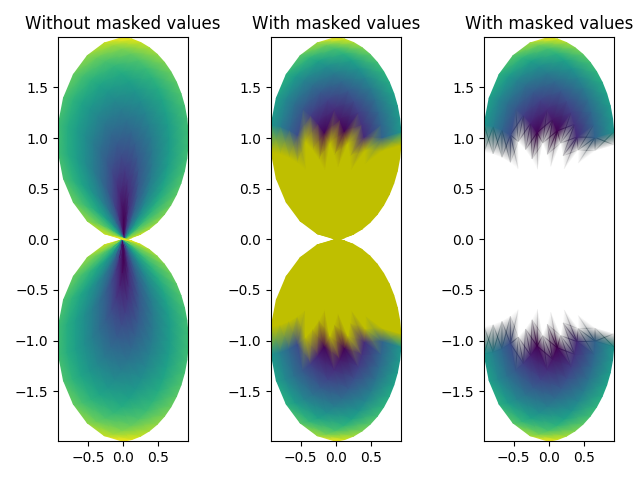### Related Topics

pcolormesh uses a QuadMesh, a faster generalization of pcolor, but with some restrictions.

import copy

from matplotlib import cm, pyplot as plt
import numpy as np

n = 12
x = np.linspace(-1.5, 1.5, n)
y = np.linspace(-1.5, 1.5, n * 2)
X, Y = np.meshgrid(x, y)
Qx = np.cos(Y) - np.cos(X)
Qz = np.sin(Y) + np.sin(X)
Z = np.sqrt(X**2 + Y**2) / 5
Z = (Z - Z.min()) / (Z.max() - Z.min())

# The color array can include masked values.
Zm = np.ma.masked_where(np.abs(Qz) < 0.5 * np.max(Qz), Z)

fig, axs = plt.subplots(nrows=1, ncols=3)

# You can control the color of the masked region. We copy the default colormap
# before modifying it.
cmap = copy.copy(cm.get_cmap(plt.rcParams['image.cmap']))